# The Lean Theorem Prover

## Many thanks to

• Cody Roux
• Georges Gonthier
• Grant Passmore
• Nikhil Swamy
• Assia Mahboubi
• Bas Spitters
• Steve Awodey
• Ulrik Buchholtz
• Tom Ball
• Parikshit Khanna

## Introduction: Lean

• New open source theorem prover
• Platform for
• Software verification & development
• Formalized mathematics
• Education (mathematics, logic, computer science)
• Synthesis (proofs & programs)
• de Bruijn's Principle: small trusted kernel
• Expressive logic
• Partial constructions: automation fills the "holes"

## Main Goal

Lean aims to bring two worlds together

• An interactive theorem prover with powerful automation
• An automated reasoning tool that
• produces (detailed) proofs,
• has a rich language,
• can be used interactively, and
• is built on a verified mathematical library

## Secondary Goals

• Minimalist and high-performace kernel
• Experiment with different flavors of type theory
• Proof irrelevant vs Proof relevant
• Impredicative vs Predicative
• Higher Inductive Types
• Quotient Types
• Observational Type Theory
• Education
• Interactive courses
• Proving should be as easy as programming
• Have Fun

## Software verification and Formalized Mathematics

• Some projects at Microsoft Research
• Disclaimer: this projects were developed before Lean existed
• They used Boogie/Z3 and Coq.

## Software verification and Formalized Mathematics

• Similar problems
• Proof stability
• Libraries are big
• Scalability issues
• Finding existing functions/theorems
• Common problems in software engineering:
• Every attempt to create a single unified language failed (ADA?)
• We keep reimplementing the same libraries over and over again
• Mixing libraries from different languages is usually a mess
• Bit rotting
• These problems also affect formalized mathematics

## What is new?

• Poweful elaboration engine that can handle
• Higher-order unification
• Definitional reductions
• Coercions
• Type classes
• Tactics

"By relieving the brain of all unnecessary work, a good notation sets it free to concentrate on more advanced problems, and in effect increases the mental power of the race." – A. N. Whitehead

## What is new?

• Poweful elaboration engine that can handle
• Small trusted kernel
• It does not contain
• Termination checker
• Fixpoint operators
• Pattern matching
• Module management

## What is new?

• Poweful elaboration engine that can handle
• Small trusted kernel
• Multi-core support
• Process theorems in parallel
• Execute/try tactics (automation) in parallel

## What is new?

• Poweful elaboration engine that can handle
• Small trusted kernel
• Multi-core support
• Fast incremental compilation

## What is new?

• Poweful elaboration engine that can handle
• Small trusted kernel
• Multi-core support
• Fast incremental compilation
• Support for mixed declarative and tactic proof style

## Dependent Type Theory

• Before we started Lean, we have studied different theorem provers: ACL2, Agda, Automath, Coq, HOL (family), Isabelle, Mizar, PVS
• Dependent type theory is really beautiful
• Bultin computational interpretation
• Same data-structure for representing proofs and terms
• Reduce code duplication, example:
• We implemented a compiler for Haskell-like recursive equations, we can use it to construct proofs by induction
• Mathematical structures (such as Groups and Rings) are first-class citizens
• Some references

## Dependent Type Theory

• Constants
bool, nat, list, tree
• Function applications
(fact n), (vector nat 10), (x + y)
• Lambda abstractions
λ x : nat, x + 1 λ (n : nat) (v : vector nat n), cons 0 v
• Function spaces
nat → nat Π (n : nat), vector nat n → vector nat (n+1)

## Dependent Type Theory

• What is the type of nat?
nat : Type
• What is the type of Type?
Type : Type

Is Lean inconsistent? NO

• Lean has a noncumulative universe hierarchy
Type.{0} : Type.{1} : Type.{2} : Type.{3} : ...
• Supports universe polymorphism
λ (A : Type.{u}) (a : A), a
• In ordinary situations you can ignore the universe parameters and simply write Type, leaving the "universe management" to Lean

## Propositions as types

• Propositions are types
∃ x : nat, x > 2
• The inhabitants/elements of a proposition P are the proofs of P
• Prop is the type of all propositions

## Architecture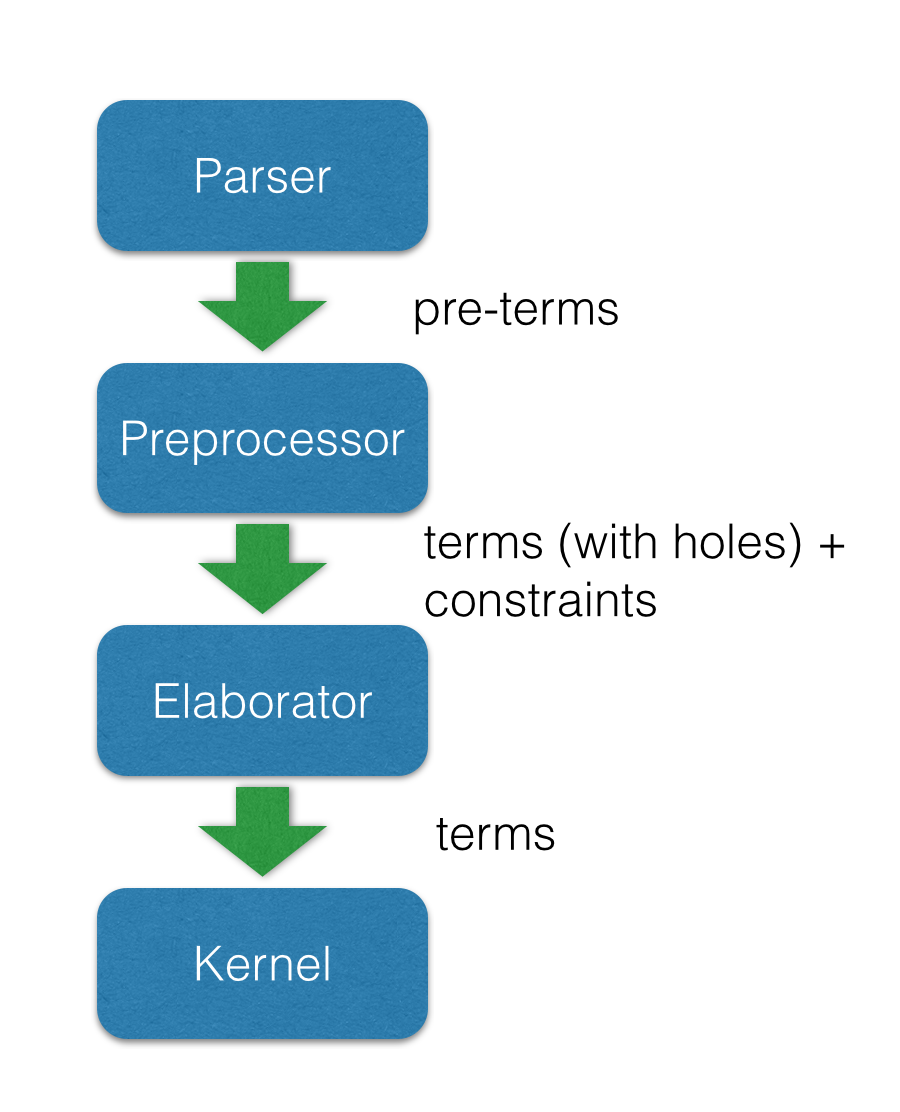## Architecture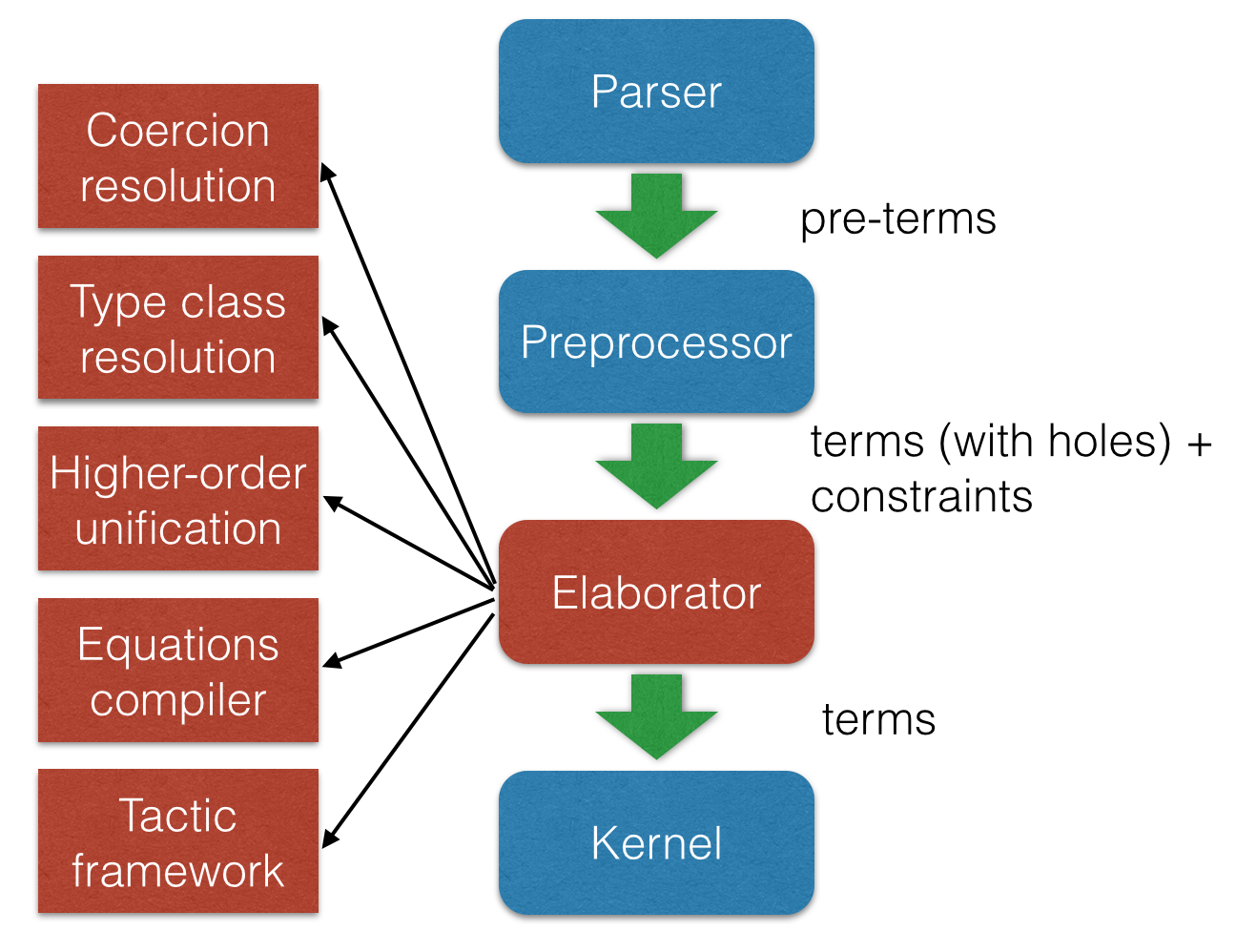## Architecture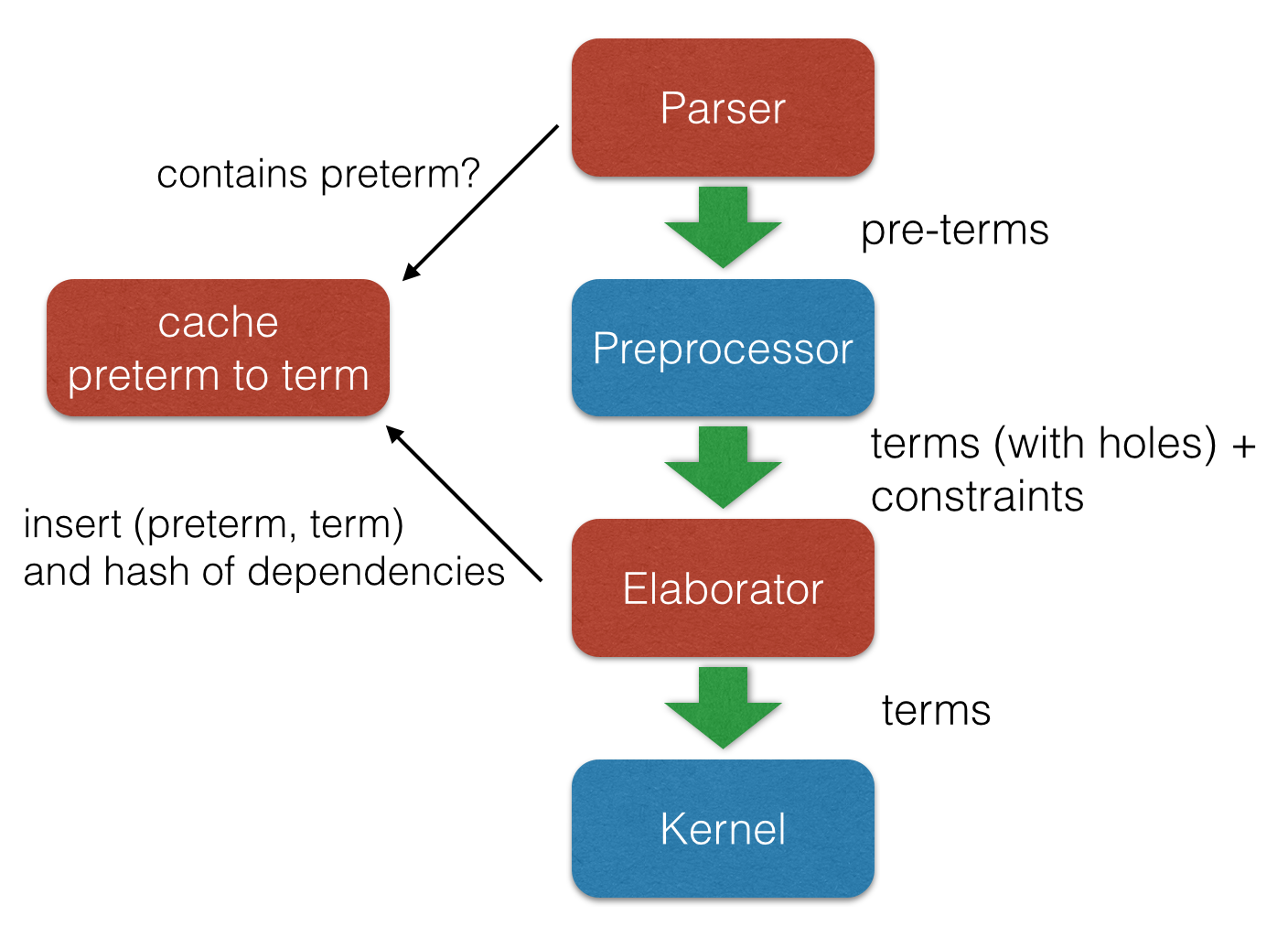## Kernel

• Kernel is implemented in two layers for easy customization
• 1st layer, dependent lambda calculus + options:
• Proof irrelevance
• Impredicative Prop
Π (x : nat), x = x -- is a Proposition ∀ (x : nat), x = x -- Alternative notation
• 2nd layer: Inductive families, Quotient types, HITs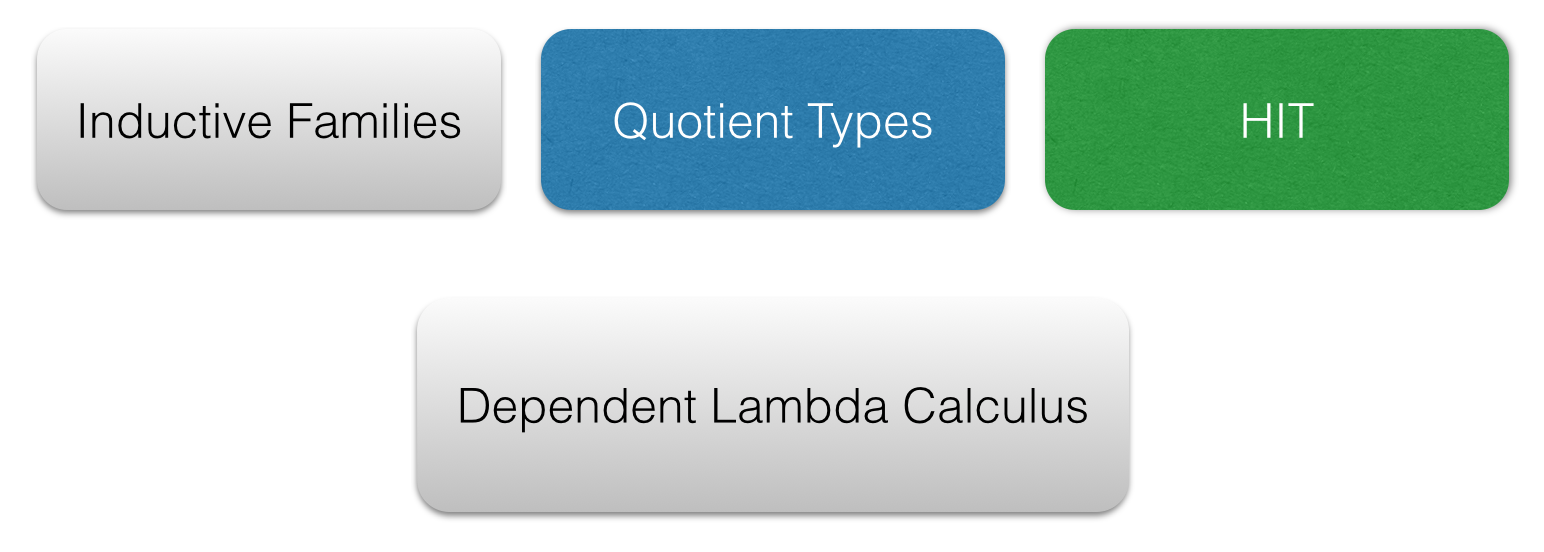## Two official libraries

• Standard
• Proof irrelevant and impredicative Prop
• Smooth transition to classical logic
• Inductive Families
• Quotient Types
• HoTT
• Proof relevant and no impredicative Prop
• Univalence axiom
• Inductive Families
• HIT
• Easy to implement experimental versions, Example: Steve Awodey asked for proof relevant and impredicative universe

## Agnostic Mathematics

• Support constructive and classical mathematics
• Computation is important to mathematics
• Core parts of the standard library are constructive
• Separation of concerns:
• Methods to write computer programs
• Freedom to use a nonconstructive theories and methods to reason about them

## Agnostic Mathematics

• Semi constructive axioms:
• Function extensionality
• Proposition extensionality
• Quotient types (implies function extensionality)
funext: (∀ x, f x = g x) → f = g propext: (p ↔ q) → p = q
• Acceptable classical axiom: proof irrelevant excluded middle
• Anti constructive: Hilbert's choice (aka magic)
• consequence: all propositions are decidable

## Freedom to trust

• Option: type check imported modules.
• Macros: semantic attachments for speeding up type checking and evaluation.
• Macros can be eliminated (expanded into pure Lean code).
• Each macro provides a function for computing the type and evaluating an instance.
• Each macro can be assigned a trust level.
• Many applications: interface with the GNU multiprecision arithmetic (GMP) library.

## Freedom to trust

• Relaxed mode
• Trust the imported modules have not been tampered
• Trust all macros
• Paranoid mode
• Retype check all imported modules (someone may have changed the binaries)
• Expand all macros (the developers may have made mistakes, GMP may be buggy)
• Stronger guarantee Retype check everything using Lean reference type checker

## Inductive families

inductive nat : Type := | zero : nat | succ : nat → nat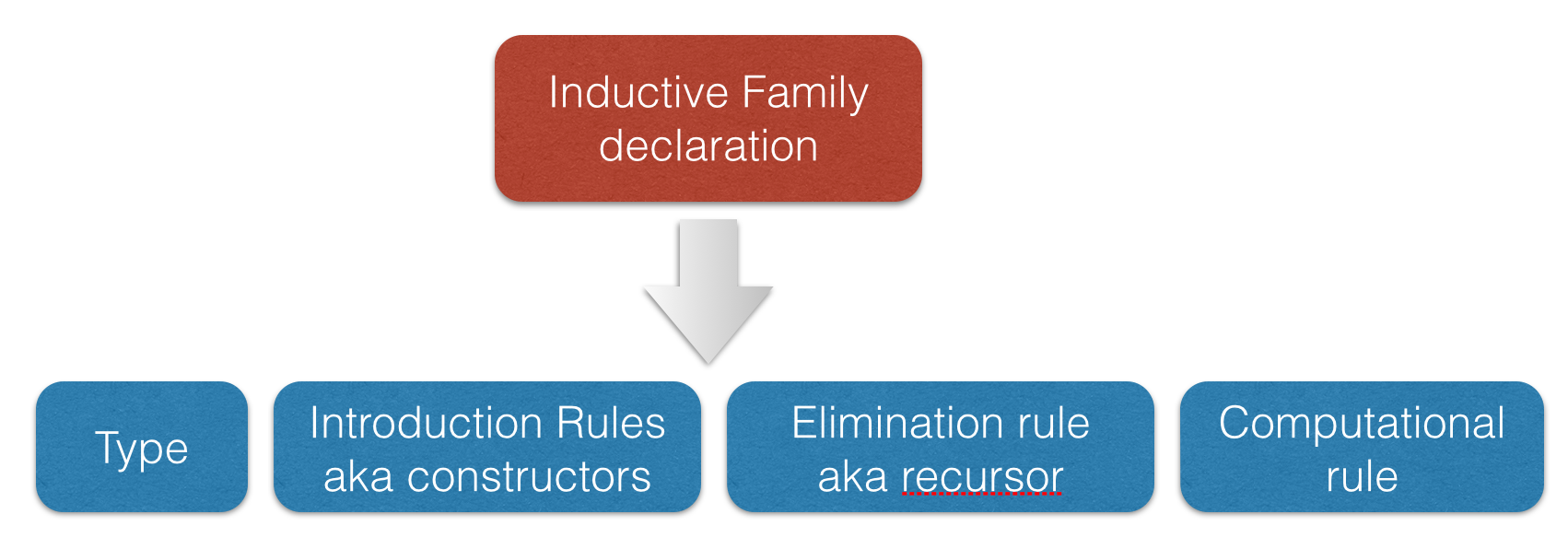nat : Type nat.zero : nat -- constructor nat.succ : nat → nat -- constructor nat.rec : Π (C : nat → Type), -- recursor C nat.zero → (Π (a : nat), C a → C (nat.succ a)) → n → C n -- Computational rules nat.rec C v f nat.zero ==> v nat.rec C v f (nat.succ n) ==> f n (nat.rec C v f n)

## Inductive families

definition add (x y : nat) : nat := nat.rec (λ n, nat) x (λ n r, succ r) y abbreviation C := λ n, nat abbreviation s := succ abbreviation z := zero abbreviation f := λ n r : nat, succ r add (s z) (s (s z)) = nat.rec C (s z) f (s (s z)) ... = s (nat.rec C (s z) f (s z)) ... = s (s (nat.rec C (s z) f z)) ... = s (s (s z))

## Inductive families

inductive vector (A : Type) : nat → Type := | nil : vector A zero | cons : Π {n}, A → vector A n → vector A (succ n)

## Inductive families

• It is possible to construct a substantial edifice of mathematics based on nothing more than the type universes, function spaces, and inductive types; everything else follows from those.

## Implicit arguments

• Curly braces indicate that argument should be inferred rather than entered explicitly.
definition id {A : Type} (a : A) : A := a check id 10 -- @id num 10 check @id num 10

## Implicit arguments

• Elaborator uses higher-order unification.
check @eq.subst -- eq.subst : ∀ {A : Type} {a b : A} {P : A → Prop}, -- a = b → P a → P b variables (A : Type) (R : A → A → Prop) variables (a b c : A) (f : A → A → A) example (H₁ : R (f a a) (f a a)) (H₂ : a = b) : R (f a b) (f b a) := eq.subst H₂ H₁ example (H₁ : R (f a a) (f a a)) (H₂ : a = b) : R (f a b) (f b a) := @eq.subst A a b (λ x : A, R (f a x) (f x a)) H₂ H₁

## Recursive equations

• Recursors are inconvenient to use.
• Compiler from recursive equations to recursors.
• Two compilation strategies: structural and well-founded recursion
definition fib : nat → nat | fib 0 := 1 | fib 1 := 1 | fib (a+2) := fib (a+1) + fib a example (a : nat) : fib (a+2) = fib (a+1) + fib a := rfl

## Recursive equations

• Proofs by induction
theorem fib_pos : ∀ n, 0 < fib n | fib_pos 0 := show 0 < 1, from zero_lt_succ 0 | fib_pos 1 := show 0 < 1, from zero_lt_succ 0 | fib_pos (a+2) := calc 0 = 0 + 0 : rfl ... < fib (a+1) + 0 : lt_right (fib_pos (a+1)) 0 ... < fib (a+1) + fib a : lt_left (fib_pos a) (fib (a+1)) ... = fib (a+2) : rfl

## Recursive equations

• Dependent pattern matching
definition map {A B C : Type} (f : A → B → C) : Π {n : nat}, vector A n → vector B n → vector C n | map nil nil := nil | map (a::va) (b::vb) := f a b :: map va vb definition zip {A B : Type} : Π {n}, vector A n → vector B n → vector (A × B) n | zip nil nil := nil | zip (a::va) (b::vb) := (a, b) :: zip va vb

## Definitional Reductions

• Elaborator must respect the computational interpretation of terms
example (A : Type) (a b : A) : (a, b).1 = a := rfl example (A B : Type) (f : A → A → B) (a : A) : (λ x, f x x) a = f a a := rfl

## Coercions

• In Lean, we can associate attributes to definitions.
• Coercion is one of the available attributes.
variable {A : Type} definition to_list [coercion] : ∀ {n : nat}, vector A n → list A | 0 nil := nil | (n+1) (a::v) := a::(to_list v) variable f : list nat → Prop variable v : vector nat 10 check f v -- f (@to_list nat 10 v)

## Namespaces

• We can group definitions, metadata (e.g., notation declarations and attributes) into namespaces.
• We can open namespaces
namespace foo definition f (a : nat) := nat.succ a eval f nat.zero end foo eval foo.f nat.zero open foo eval f nat.zero open nat check 1 + 2 open nat (hiding add sub) open nat (renaming add → nadd) open nat (rec_on) open [notations] nat open -[classes] nat

notation a + b := add a b notation a + b := bor a b eval 1 + 2 eval tt + ff
• We can use namespaces to avoid unwanted ambiguity.
check (#nat a + b)

import algebra.category open eq.ops category functor natural_transformation variables {ob₁ ob₂ : Type} {C : category ob₁} {D : category ob₂} {F G H : C ⇒ D} definition nt_compose (η : G ⟹ H) (θ : F ⟹ G) : F ⟹ H := natural_transformation.mk (take a, η a ∘ θ a) (take a b f, calc H f ∘ (η a ∘ θ a) = (H f ∘ η a) ∘ θ a : assoc ... = (η b ∘ G f) ∘ θ a : naturality ... = η b ∘ (G f ∘ θ a) : assoc ... = η b ∘ (θ b ∘ F f) : naturality ... = (η b ∘ θ b) ∘ F f : assoc

## Type classes

• Synthesis procedure based on lambda-Prolog
• Big picture
• Mark some inductive families as classes
• Mark some definitions as (generators of) instances
• Indicate that some implicit arguments must be synthesized using type classes
• Instances are treated as Horn clauses

## Inhabited Type Class

inductive inhabited [class] (A : Type) : Type := mk : A → inhabited A definition default (A : Type) [h : inhabited A] : A := inhabited.rec (λ a, a) h definition prop_inhabited [instance] : inhabited Prop := inhabited.mk true definition nat_inhabited [instance] : inhabited nat := inhabited.mk nat.zero definition fun_inhabited [instance] (A B : Type) [h : inhabited B] : inhabited (A → B) := inhabited.mk (λ x : A, default B) definition prod_inhabited [instance] (A B : Type) [ha : inhabited A] [hb : inhabited B] : inhabited (A × B) := inhabited.mk (default A, default B) eval default (nat → nat × Prop) -- ⇒ λ (a : ℕ), (0, true)

## Decidable Type Class

• An element of Prop is said to be

decidable if we can decide whether it is true or false.

inductive decidable [class] (p : Prop) : Type := | inl : p → decidable p | inr : ¬p → decidable p
• Having an element t : decidable p is stronger than having an element t : p ∨ ¬p
• The expression if c then t else e contains an implicit argument [d : decidable c].
• If Hilbert's choice is imported, then all propositions are decidable (smooth transition to classical reasoning).

## Decidable Type Class

definition ball (n : nat) (P : nat → Prop) : Prop := ∀ x, x < n → P x definition dec_ball [instance] [H : decidable_pred P] : Π (n : nat), decidable (ball n P) | dec_ball 0 := inl (ball_zero P) | dec_ball (a+1) := match dec_ball a with | inl iH := match H a with | inl Pa := inl (ball_succ_of_ball iH Pa) | inr nPa := inr (not_ball_of_not nPa) end | inr niH := inr (not_ball_succ_of_not_ball niH) end definition is_constant_range (f : nat → nat) (n : nat) : bool := if ∀ i, i < n → f i = f 0 then tt else ff

## Tactics

• Automation such as rewrite engined, simplifiers and decision procedures are integrated into the system as tactics.
• A placeholder/hole can be viewed as a goal
• A proof state is a sequence of goals, substitution (already solved holes), and postponed constraints.
• A tactic is a function from proof state to a lazy stream of proof states (very similar to Isabelle).
• Tacticals are tactic combinadors: andthen, orelse, par, …

## Tactics

• We can switch to tactic mode using begin … end or by …
example (p q : Prop) (Hp : p) (Hq : q) : p ∧ q ∧ p := begin apply and.intro, exact Hp, apply and.intro, exact Hq, exact Hp end example (H₁ : a + 0 = 0) (H₂ : b + 0 = 0) : a + b + 0 = 0 := begin rewrite add_zero at *, rewrite [H₁, H₂] end

## Structures

• Special kind of inductive datatype (only one constructor)
• Projections are generated automatically
• "Inheritance"
• Extensively used to formalize the algebraic hierarchy
• We can view them as parametric modules
structure has_mul [class] (A : Type) := (mul : A → A → A) structure semigroup [class] (A : Type) extends has_mul A := (mul_assoc : ∀a b c, mul (mul a b) c = mul a (mul b c)) ... structure group [class] (A : Type) extends monoid A, has_inv A := (mul_left_inv : ∀a, mul (inv a) a = one)

## Structures

structure ring [class] (A : Type) extends add_comm_group A, monoid A, distrib A variable {A : Type} theorem ring.mul_zero [s : ring A] (a : A) : a * 0 = 0 := have H : a * 0 + 0 = a * 0 + a * 0, from calc a * 0 + 0 = a * 0 : by rewrite add_zero ... = a * (0 + 0) : by rewrite add_zero ... = a * 0 + a * 0 : by rewrite left_distrib, show a * 0 = 0, by rewrite -(add.left_cancel H)

structure ring [class] (A : Type) extends add_comm_group A, monoid A, distrib A variable {A : Type} theorem ring.mul_zero [s : ring A] (a : A) : a * 0 = 0 := ... theorem ring.zero_mul [s : ring A] (a : A) : 0 * a = 0 := ... definition ring.to_semiring [instance] [s : ring A] : semiring A := ⦃ semiring, s, mul_zero := ring.mul_zero, zero_mul := ring.zero_mul ⦄

## Structures (concrete instances)

protected definition int.linear_ordered_comm_ring [instance] : algebra.linear_ordered_comm_ring int := ⦃algebra.linear_ordered_comm_ring, int.integral_domain, le := int.le, le_refl := int.le.refl, le_trans := int.le.trans, le_antisymm := int.le.antisymm, lt := int.lt, le_of_lt := int.le_of_lt, lt_irrefl := int.lt.irrefl, ... le_iff_lt_or_eq := int.le_iff_lt_or_eq, le_total := int.le.total, zero_ne_one := int.zero_ne_one, zero_lt_one := int.zero_lt_one, add_lt_add_left := int.add_lt_add_left⦄
• Is int a add_group? Yes
int.linear_ordered_comm_ring : algebra.linear_ordered_comm_ring int linear_ordered_comm_ring int → comm_ring int comm_ring int → ring int ring int → add_comm_group int add_comm_group int → add_group int

## Sylow theorem

variables {A : Type} [ambA : group A] [finA : fintype A] [deceqA : decidable_eq A] include ambA deceqA finA theorem cauchy_theorem : ∀ p, prime p → p ∣ card(A) → ∃ g : A, order(g) = p theorem first_sylow_theorem : ∀ p, prime p → ∀ n, p^n ∣ card(A) → ∃ (H : finset A) (sg : is_finsubg H), card(H) = p^n

## Bundled structures

structure Semigroup : Type := (carrier : Type) (mul : carrier → carrier → carrier) (infix * := mul) (mul_assoc : ∀ a b c : carrier, (a * b) * c = a * (b * c)) notation a * b := Semigroup.mul _ a b attribute Semigroup.carrier [coercion] structure morphism (S1 S2 : Semigroup) : Type := (mor : S1 → S2) (resp_mul : ∀ a b : S1, mor (a * b) = (mor a) * (mor b)) attribute morphism.mor [coercion] example (S1 S2 : Semigroup) (f : morphism S1 S2) (a : S1) : f (a * a * a) = f a * f a * f a := calc f (a * a * a) = f (a * a) * f a : morphism.resp_mul f ... = f a * f a * f a : morphism.resp_mul f

## Future work

• Auto tactic based on equational reasoning, matching, heuristic instantiation, …
• Decision procedures for arithmetic
• Efficient evaluator
• Better support for proof by reflection
• Better libraries (ongoing work)